Industrial Engineering - 2

# Industrial Engineering - 2

Test Description

## 20 Questions MCQ Test GATE Mechanical (ME) 2023 Mock Test Series | Industrial Engineering - 2

Industrial Engineering - 2 for Mechanical Engineering 2023 is part of GATE Mechanical (ME) 2023 Mock Test Series preparation. The Industrial Engineering - 2 questions and answers have been prepared according to the Mechanical Engineering exam syllabus.The Industrial Engineering - 2 MCQs are made for Mechanical Engineering 2023 Exam. Find important definitions, questions, notes, meanings, examples, exercises, MCQs and online tests for Industrial Engineering - 2 below.
Solutions of Industrial Engineering - 2 questions in English are available as part of our GATE Mechanical (ME) 2023 Mock Test Series for Mechanical Engineering & Industrial Engineering - 2 solutions in Hindi for GATE Mechanical (ME) 2023 Mock Test Series course. Download more important topics, notes, lectures and mock test series for Mechanical Engineering Exam by signing up for free. Attempt Industrial Engineering - 2 | 20 questions in 60 minutes | Mock test for Mechanical Engineering preparation | Free important questions MCQ to study GATE Mechanical (ME) 2023 Mock Test Series for Mechanical Engineering Exam | Download free PDF with solutions
 1 Crore+ students have signed up on EduRev. Have you?
Industrial Engineering - 2 - Question 1

### Match the following numbers with the letters given below by using the cods given below: 1. A continuum describing the degree to which a system’s components may be separated and recombined. 2. The ability to move and change direction and position of the body quickly and effectively while under control. 3. The ability to withstand its variations in demand. 4. The product/part which can be usable even at its worst stage. p. Modularity q. Flexibility r. Robust s. Agility

*Answer can only contain numeric values
Industrial Engineering - 2 - Question 2

### An industrial machine tools manufacturer suppliers replacements from its inventory. For a particular part, the annual demand is expected to be 750 units. Machine setup costs are \$50, carrying costs are 25 percent per year, and the part is valued in inventory at \$35 each. The economic order quantity is __________ UNITS.

Detailed Solution for Industrial Engineering - 2 - Question 2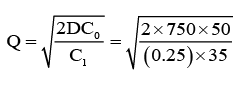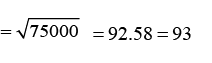Industrial Engineering - 2 - Question 3

### The parts are processing on the machines launched into the system in the order of their arrivals

Industrial Engineering - 2 - Question 4

“The liner programming which starts with the optimal solution and infeasible solution, after some successive iteration, it will move to the optimal solution and the feasible” is

Detailed Solution for Industrial Engineering - 2 - Question 4

The crux of the Dual simplex method is to start with the better than optimal and infeasible basic solution. The optimality and the feasibility conditions are designed to preserve the optimality and the feasibility of the basic solutions while moving the solution iterations toward feasibility.

Industrial Engineering - 2 - Question 5

A dummy activity is used in PERT network to describe

Industrial Engineering - 2 - Question 6

Follow the symmetrical relation between primal and dual problems, assuming the primal to be a maximization problem,

(a) Number of variables in the primal equal to the number of constraints in the dual.

(b) Less than or equal to constraint in the primal equal to non-negative variable in the dual.

(c) Unrestricted variable in the primal equal to type constraint.

Among the above statements:

Industrial Engineering - 2 - Question 7

NC contouring is an example of

Industrial Engineering - 2 - Question 8

The 10-inch sine bar is used to measure the angularity of the taper on the shaft positioned between two bench centers. The difference in height (H 2 – H 1 ) over the buttons is measured using a Vernier height gauge and a dial test indicator. Calculate the included angle of the taper when H 2 = 6.122 inches and H 1 4.678 inches.

Detailed Solution for Industrial Engineering - 2 - Question 8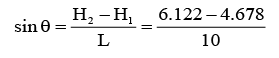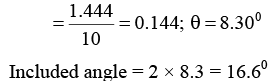*Answer can only contain numeric values
Industrial Engineering - 2 - Question 9

In a 3-wire inspection of metric external thread of M16 × 2, the measurement over the wires were found to be 16.655 mm. Ten the effective pitch diameter of the thread is ……….. mm.

Detailed Solution for Industrial Engineering - 2 - Question 9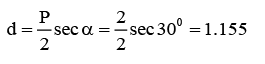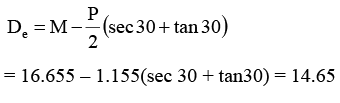Industrial Engineering - 2 - Question 10

Match List – I with List – II and select the correct answers using the codes given below the lists: List – I

P. CNC
Q. DNS
R. FMS
S. Automatic lathe

List – II

1. Simultaneous control of several machine tools

2. Mass production of a single variety of parts

3. Flexible control of a single machine tool

4. Batch projection of a family of parts

*Answer can only contain numeric values
Industrial Engineering - 2 - Question 11

Demand for videotapes of a financial TB program varies uniformly between 500 and 900 copies. The distributor pays Rs. 4 per tape and realizes Rs. 18 income on each sale. Unsold tapes cannot be returned and have no salvage value. Then the optimum stocking level is ___________.

Detailed Solution for Industrial Engineering - 2 - Question 11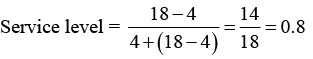Stocking level = 500 + 0.8 (900 – 500) = 500 + 320 = 820

Industrial Engineering - 2 - Question 12

A repairman is to be hired by a company to repair machines whose break-down follow a Poisson process, with an average rate of four per hour. The cost of non-productive machine time is Rs. 90 per hour. The company has the option of choosing either a fast or slow repairman. The fast repairman (F) charges Rs. 70 per hour and will repair machines at an average rate of 7 per hour. While the slow repairman charges Rs. 50 per hour and repairs machines at an average rate of Rs. 6 per hour. Which repairman should be hired?

Detailed Solution for Industrial Engineering - 2 - Question 12

Fast repairman Total wage = 70 × 8 = 560

Expected number of machinery in system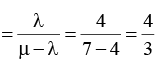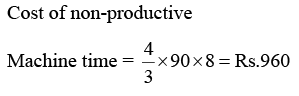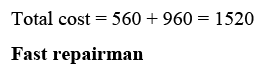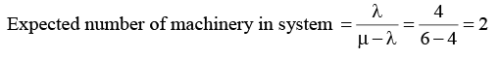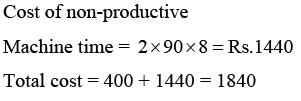Industrial Engineering - 2 - Question 13

Demand averages 50 units per week with a standard deviation f 20 units per week; lead time is constant at 1 week (constant lead time with LT < order cycle). Then find the re-order point (ROP) for a service level of 98% from normal curve Z = 2.05 for 48%

Detailed Solution for Industrial Engineering - 2 - Question 13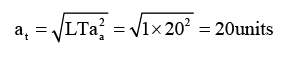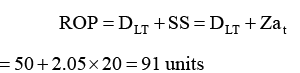Industrial Engineering - 2 - Question 14

Consider the following statements: 1. ABC analysis is based on Pareto’s principal 2. MRP and MPS are the inputs for aggregate planning and control 3. Simulation can be used for inventory control 4. EOQ formula ignores variations in demand pattern

Detailed Solution for Industrial Engineering - 2 - Question 14

ABC- Pareto → True

MRP, MPS – Aggregation → True

Simulation – Inventory → True

EOQ ignores variations in demand pattern → True

→ In the inventory A-class, B-class and C-class items were divided according to their nature. It was implemented by the scientist Advert Pareto an Italian based on 80:20 principle.

→ Material requirement planning in the Inp0ut for master-production schedule and the combination of MPS and MRP are inputs for aggregation production and planning.

→ In the inventory simulation can be done up to some extent.

→ Economic order quantity is very well applicable for the demands which are constant in demand.

Industrial Engineering - 2 - Question 15

There are five jobs, each of which must go through the two machines A and B in the order AB. Processing times are given below:

Processing time (hours)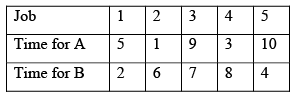By following the sequence procedure, calculate the total idle time for machine A and machine B respectively.

Detailed Solution for Industrial Engineering - 2 - Question 15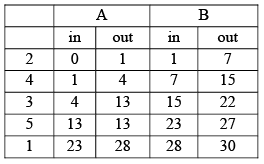Idle time for A → (28 to 30hrs ) = 2hrs Idle time for B → (0 − 1,22 − 23,27 − 28) = 3hrs

Industrial Engineering - 2 - Question 16

Arrivals at a telephone booth are considered to be Poisson, with an average time of 10 minutes between one arrival and the next. The length of the phone call is assumed to be distributed exponentially, with mean time 3 minutes. Find the probability that an arrival finds that four persons are waiting for their turn.

Detailed Solution for Industrial Engineering - 2 - Question 16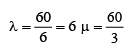customers/hair;  = 20 customers/hair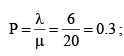four people waiting for their turn in the queue implies a total of 5 people in the system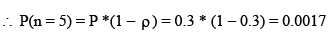Industrial Engineering - 2 - Question 17

A farm machinery manufacture has an output rate of 320 hours per week and has measured the load on his shop as follows:

Work in Process:

Current Requirement – 960 hours

Long term orders – 320 hours

Then what is the manufacture lead time?

Detailed Solution for Industrial Engineering - 2 - Question 17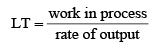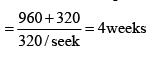Industrial Engineering - 2 - Question 18

A company has 3 plants and four ware houses. The supply and demand in units and the corresponding transpiration costs are given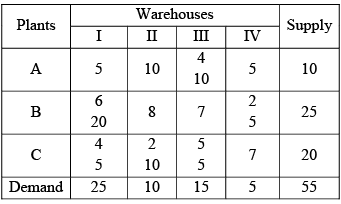Q.

Choose the correct statement

Detailed Solution for Industrial Engineering - 2 - Question 18

The solution is not degenerate, because the number of occupied cells is not less than the required number of m + n – 1

Solution is optimal because all the ∆q's

The total cost involved is Rs. 235

Industrial Engineering - 2 - Question 19

The parts are processing on the machines launched into the system in the order of their arrivals

Industrial Engineering - 2 - Question 20

If the cost for the route B-III is reduced form Rs. 7 to Rs. 6 per unit, what will be the total cost?

Detailed Solution for Industrial Engineering - 2 - Question 20

If the cost per unit for the rate B-III equal to 6, it would have ∆ id = +1 and hence optimal solution obtained is x13 = 10; x 21 = 15; x 23 = 5; x 24 = 5; x 31 = 10 and x 32 = 10

Total cost = Rs. 230

## GATE Mechanical (ME) 2023 Mock Test Series

26 docs|239 tests
 Use Code STAYHOME200 and get INR 200 additional OFF Use Coupon Code
Information about Industrial Engineering - 2 Page
In this test you can find the Exam questions for Industrial Engineering - 2 solved & explained in the simplest way possible. Besides giving Questions and answers for Industrial Engineering - 2, EduRev gives you an ample number of Online tests for practice

## GATE Mechanical (ME) 2023 Mock Test Series

26 docs|239 tests